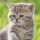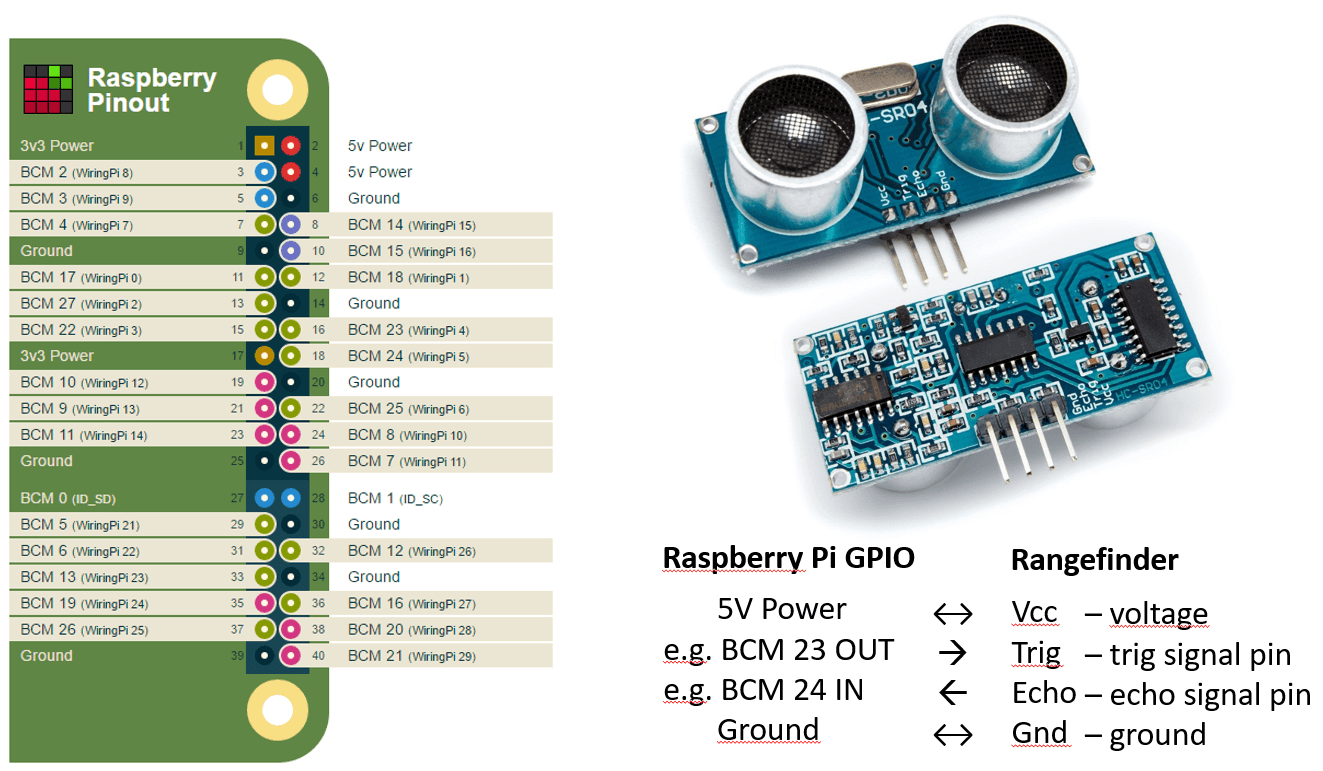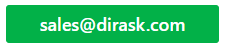Home
IT Knowledge
IT Career
Inspiration
Languages
EN

# Raspberry PI - how to use ultrasonic rangefinder with c++ (cpp)?

4 points
Created by:Kate_C
19790

## 1. Connect rangefinder to Raspberry PI:Raspberry PI B+

``````\$ wget abyz.me.uk/rpi/pigpio/pigpio.zip
\$ unzip pigpio.zip
\$ cd PIGPIO
\$ make
\$ sudo make install
\$ rm pigpio.zip
\$ sudo rm -rf PIGPIO``````

## 3. Create `program.cpp` file:

``````#include <pigpio.h>
#include <iostream>
#include <time.h>

#include <stdlib.h>
#include <sys/time.h>

using namespace std;

struct timeval tv;

double getTime()
{
gettimeofday(&tv, NULL);

return (double)tv.tv_sec + (double)tv.tv_usec * 0.000001;
}

// https://en.wikipedia.org/wiki/Speed_of_sound
int coefficient = 17150; // it should be selected experimentally - it is half of speed of sound in air in cm/s

int TRIG = 23; // BCM/GPIO pin number
int ECHO = 24; // BCM/GPIO pin number

void initializePins()
{
gpioSetMode(TRIG, PI_OUTPUT);
gpioSetMode(ECHO, PI_INPUT);
}

bool waitValue(int value, int limit = 10000) // use 1000000 to increase measurement distance
{
for(int i = 0; gpioRead(ECHO) == value; ++i)
{
if(i >= limit)
return false;
}

return true;
}

double detectDistance()
{
gpioWrite(TRIG, 0); // reset rangefinder state
usleep(500000); // wait for reset state - use 100000 to speedup program
gpioWrite(TRIG, 1); // hi state runs rangefinder measurement
usleep(10); // 10 us of hi level is necessary to start measurement - depended on rangefinder model
gpioWrite(TRIG, 0); // prepare rangefinder for measurement

if(waitValue(0)) // wait until lo level is achieved on echo pin
{
double pulseStart = getTime();

if(waitValue(1)) // wait until hi level is achieved on echo pin - hi level duration is proportional to distance
{
double pulseEnd = getTime();

double duration = pulseEnd - pulseStart;
double distance = duration * coefficient;

return distance;
}
}

cout << "Measurement error!";

// Troubleshooting:
// - check correctness of pin connection,
// - check supply in case of many devices connected,
// - check maximal supported distance by rangefinder

return 0.0 / 0.0;
}

int main ()
{
if(gpioInitialise() < 0)
cout << "PIGPIO initialisation failure!" << endl;

else
{
cout << "PIGPIO initialisation success!" << endl;

initializePins();

for(int i = 0; i < 100; ++i) // for example: 100 measurements
{
double distance = detectDistance();

cout << "Distance: " << distance << "cm" << endl;
}

gpioTerminate();
}

return 0;
}``````

## 4. Complile program

``\$ gcc -o program -lpigpio -lpthread -lrt program.cpp``

## 5. Run program

``\$ ./program``

Note: be sure that program has execution permissions; otherwise run:

``\$ chmod +x program``

## 6. For QtCreator IDE pro file configuration can be used:

``````TEMPLATE = app
CONFIG += console
CONFIG -= qt

SOURCES += program.cpp``````